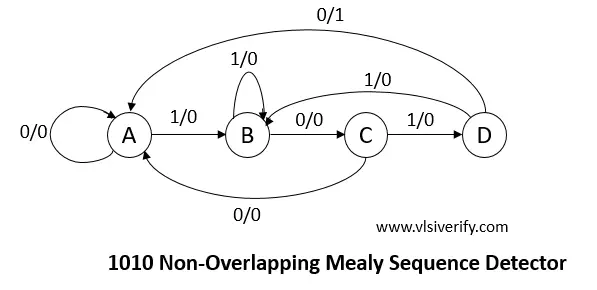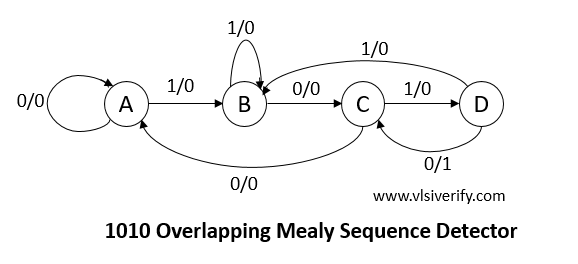Tutorials

In mealy machine, output depends on the present state and current input. It is important to understand basics of finite state machine (FSM) and sequence detector

## 1010 non-Overlapping Mealy Sequence Detector Verilog Code``````module seq_detector_1010(input bit clk, rst_n, x, output z);
parameter A = 4'h1;
parameter B = 4'h2;
parameter C = 4'h3;
parameter D = 4'h4;

bit [3:0] state, next_state;
always @(posedge clk or negedge rst_n) begin
if(!rst_n) begin
state <= A;
end
else state <= next_state;
end

always @(state or x) begin
case(state)
A: begin
if(x == 0) next_state = A;
else       next_state = B;
end
B: begin
if(x == 0) next_state = C;
else       next_state = B;
end
C: begin
if(x == 0) next_state = A;
else       next_state = D;
end
D: begin
if(x == 0) next_state = A; //This state only differs when compared with Mealy Overlaping Machine
else       next_state = B;
end
default: next_state = A;
endcase
end
assign z = (state == D)? 1:0;
endmodule``````

## Testbench Code

``````module TB;
reg clk, rst_n, x;
wire z;

seq_detector_1010 sd(clk, rst_n, x, z);
initial clk = 0;
always #2 clk = ~clk;

initial begin
\$monitor("%0t: x = %0b, z = %0b", \$time, x, z);
x = 0;
#1 rst_n = 0;
#2 rst_n = 1;

#3 x = 1;
#4 x = 1;
#4 x = 0;
#4 x = 1;
#4 x = 0;
#4 x = 1;
#4 x = 0;
#4 x = 1;
#4 x = 0;
#4 x = 1;
#4 x = 0;
#10;
\$finish;
end

initial begin
// Dump waves
\$dumpfile("dump.vcd");
\$dumpvars(0);
end
endmodule``````

Output:

``````0: x = 0, z = 0
6: x = 1, z = 0
14: x = 0, z = 0
18: x = 1, z = 0
22: x = 0, z = 1
26: x = 1, z = 0
30: x = 0, z = 0
34: x = 1, z = 0
38: x = 0, z = 1
42: x = 1, z = 0
46: x = 0, z = 0``````

## 1010 Overlapping Mealy Sequence Detector Verilog Code``````module seq_detector_1010(input bit clk, rst_n, x, output z);
parameter A = 4'h1;
parameter B = 4'h2;
parameter C = 4'h3;
parameter D = 4'h4;

bit [3:0] state, next_state;
always @(posedge clk or negedge rst_n) begin
if(!rst_n) begin
state <= A;
end
else state <= next_state;
end

always @(state or x) begin
case(state)
A: begin
if(x == 0) next_state = A;
else       next_state = B;
end
B: begin
if(x == 0) next_state = C;
else       next_state = B;
end
C: begin
if(x == 0) next_state = A;
else       next_state = D;
end
D: begin
if(x == 0) next_state = C;
else       next_state = B;
end
default: next_state = A;
endcase
end
assign z = (state == D)? 1:0;
endmodule``````

## Testbench Code

``````module TB;
reg clk, rst_n, x;
wire z;

seq_detector_1010 sd(clk, rst_n, x, z);
initial clk = 0;
always #2 clk = ~clk;

initial begin
\$monitor("%0t: x = %0b, z = %0b", \$time, x, z);
x = 0;
#1 rst_n = 0;
#2 rst_n = 1;

#3 x = 1;
#4 x = 1;
#4 x = 0;
#4 x = 1;
#4 x = 0;
#4 x = 1;
#4 x = 0;
#4 x = 1;
#4 x = 0;
#4 x = 1;
#4 x = 0;
#10;
\$finish;
end

initial begin
// Dump waves
\$dumpfile("dump.vcd");
\$dumpvars(0);
end
endmodule``````

Output:

``````0: x = 0, z = 0
6: x = 1, z = 0
14: x = 0, z = 0
18: x = 1, z = 0
22: x = 0, z = 1
26: x = 1, z = 0
30: x = 0, z = 1
34: x = 1, z = 0
38: x = 0, z = 1
42: x = 1, z = 0
46: x = 0, z = 1
50: x = 0, z = 0``````

Verilog Codes